Name:    Chapter 9 Practice MC Test: Testing a Claim

Multiple Choice
Identify the choice that best completes the statement or answers the question.

1.

DDT is an insecticide that accumulates up the food chain. Predator birds can be contaminated with quite high levels of the chemical by eating many lightly contaminated prey. One effect of DDT upon birds is to inhibit the production of the enzyme carbonic anhydrase, which controls calcium metabolism. It is believed that this causes eggshells to be thinner and weaker than normal and makes the eggs more prone to breakage. (This is one of the reasons why the condor in California is near extinction.) An experiment was conducted where 16 sparrow hawks were fed a mixture of 3 ppm dieldrin and 15 ppm DDT (a combination often found in contaminated prey). The first egg laid by each bird was measured, and the mean shell thickness was found to be 0.19 mm. A “normal” eggshell has a mean thickness of 0.2 mm.

The null and alternative hypotheses are
 A)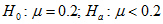B)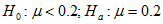C)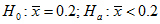D)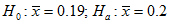E)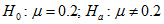2.

A significance test allows you to reject a hypothesis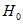in favor of an alternative Ha at the 5% level of significance.  What can you say about significance at the 1% level?
 A)can be rejected at the 1% level of significance. B) There is insufficient evidence to rejectat the 1% level of significance. C) There is sufficient evidence to acceptat the 1% level of significance. D) Ha can be rejected at the 1% level of significance. E) The answer can’t be determined from the information given.

3.

In a test of H0: µ = 100 against Ha: µ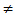100, a sample of size 10 produces a sample mean of 103 and a P-value of 0.08. Thus, at the 0.05 level of significance
 A) there is sufficient evidence to conclude that µ100. B) there is sufficient evidence to conclude that µ = 100. C) there is insufficient evidence to conclude that µ = 100. D) there is insufficient evidence to conclude that µ100. E) there is sufficient evidence to conclude that µ = 103.

4.

Which of the following is not a condition for performing inference about a population mean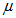?
 A) Inference is based on n independent measurements. B) The population distribution is Normal or the sample size is large (say n > 30). C) The sample size must be less than 10% of the population size. D) The data are obtained from an SRS from the population of interest. E) Both np and n(1 – p) are 10 or greater.

5.

Resting pulse rate is an important measure of the fitness of a person's cardiovascular system, with a lower rate indicative of greater fitness. The mean pulse rate for all adult males is approximately 72 beats per minute. A random sample of 25 male students currently enrolled in the Faculty of Agriculture was selected and the mean resting pulse rate was found to be 80 beats per minute with a standard deviation of 20 beats per minute. The experimenter wishes to test if the students are less fit, on average, than the general population.

A possible Type II error here would be to
 A) conclude that the students are less fit (on average) than the general population when in fact they have equal fitness on average. B) conclude that the students have the same fitness (on average) as the general population when in fact they are less fit (on average). C) conclude that the students have the same fitness (on average) as the general population when in fact they have the same fitness (on average). D) conclude that the students are less fit (on average) than the general population, when, in fact, they are less fit (on average). E) conclude that the students have the same fitness (on average) when in fact they are more fit (on average).

6.

Resting pulse rate is an important measure of the fitness of a person's cardiovascular system, with a lower rate indicative of greater fitness. The mean pulse rate for all adult males is approximately 72 beats per minute. A random sample of 25 male students currently enrolled in the Faculty of Agriculture was selected and the mean resting pulse rate was found to be 80 beats per minute with a standard deviation of 20 beats per minute. The experimenter wishes to test if the students are less fit, on average, than the general population.

A possible Type I error here would be to
 A) conclude that the students are less fit (on average) than the general population when in fact they have equal fitness on average. B) conclude that the students have the same fitness (on average) as the general population when in fact they are less fit (on average). C) conclude that the students have the same fitness (on average) as the general population when in fact they have the same fitness (on average). D) conclude that the students are less fit (on average) than the general population, when, in fact, they are less fit (on average). E) conclude that the students have the same fitness (on average) when in fact they are more fit (on average).

7.

Which of the following conditions are not necessary for the use of the one-proportion z procedures?
 A) The sample size is at least 30 B) the data must be a random sample from the population of interest C) The sample size is less than 10% of the population size D)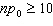E)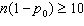8.

You are thinking of using a t-procedure to test hypotheses about the mean of a population using a significance level of 0.05. You know the population distribution is normal. Which of the following statements is correct?
 A) You should not use the t-procedure here since the populatiuon mean is less than 30. B) You may use the t-procedure here provided you have a sample size that is 30 or greater. C) You may use the t-procedure here with any sample size. D) You should not use the t-procedure here because this is a problem of proportions E) You may use the t-procedure here provided there are no outliers or strong skewness in the sample data.

9.

An educational researcher is collecting data for a study on the job market for graduating business majors in 2012. One variable of interest is the percentage of business grads who were able to find employment in their field within 6 months of obtaining their degree. The researcher hypothesizes in advance of the study that less than 40% of business grads will be able to find jobs within 6 months of graduation. To answer this question, one should use
 A) one-proportion z test B) one-sample t test C) one-sample z test D) one-proportion t test E) none of the above

10.

A random sample survey of 175 skiers at a large European resort found that 28% of the those surveyed had taken a ski vacation outside their own country within the past year. The observed value of the test statistic for testing the null hypothesis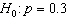versus the alternative hypothesis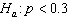is
 A)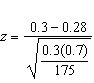B)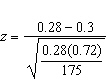C)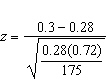D)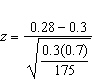E) None of the above